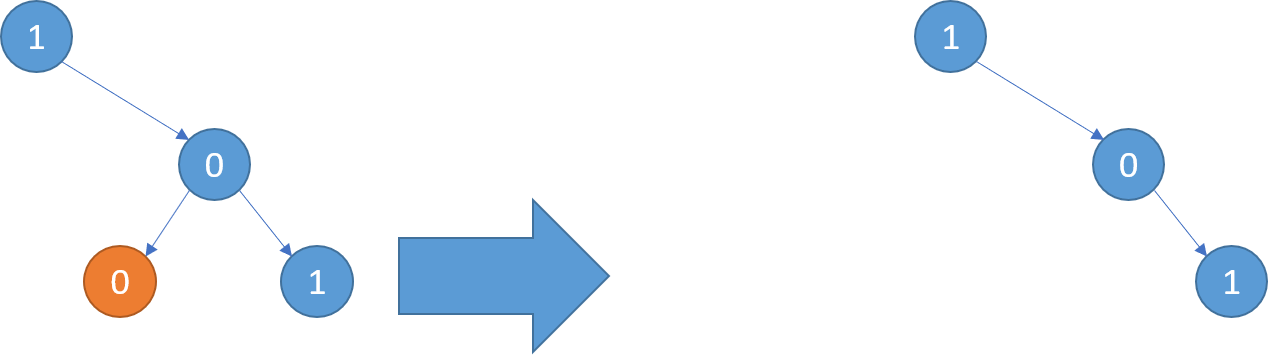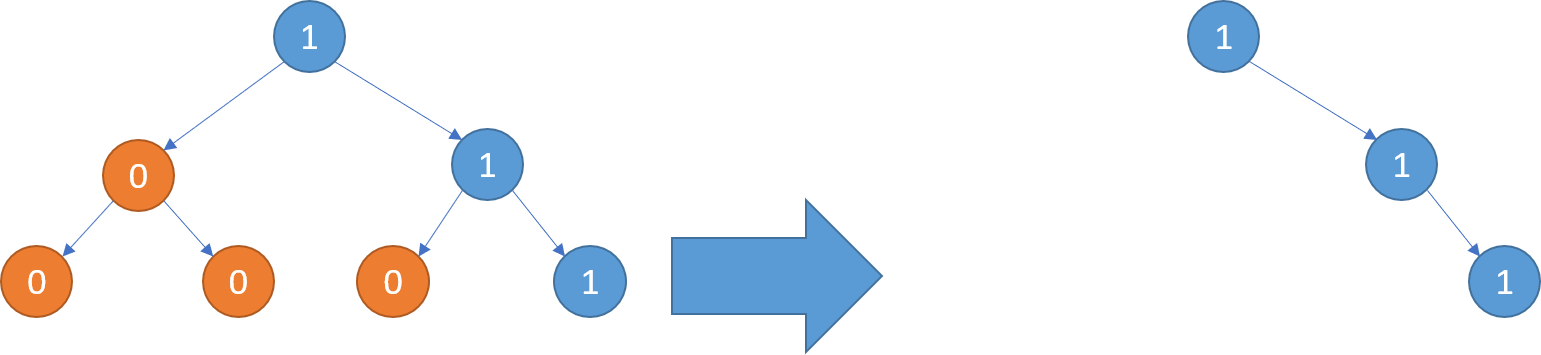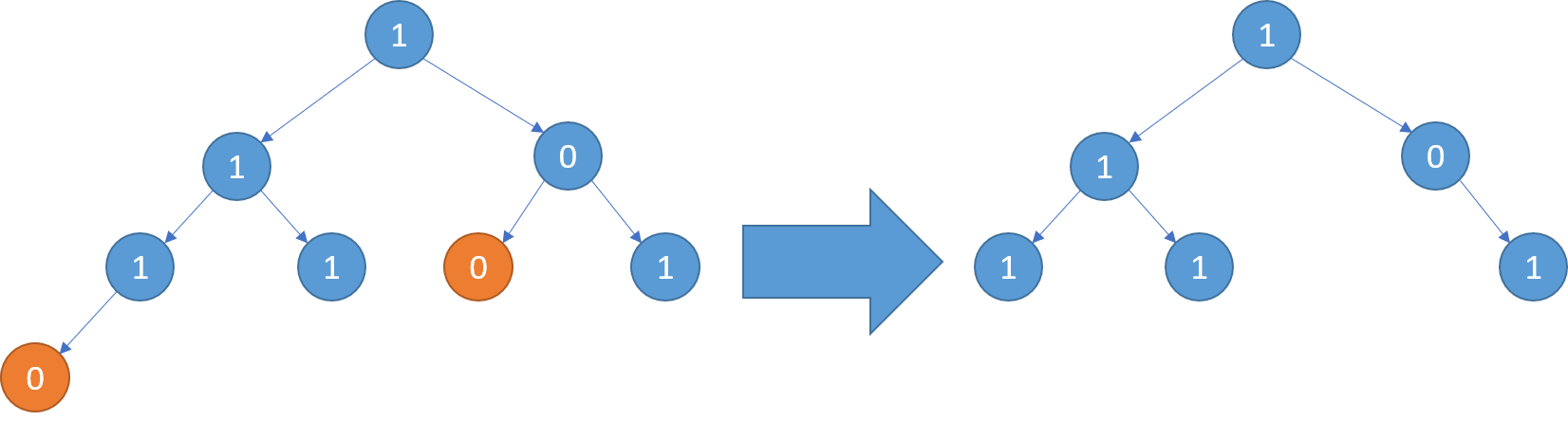## 题目描述：

LeetCode 814. Binary Tree Pruning

We are given the head node `root` of a binary tree, where additionally every node's value is either a 0 or a 1.

Return the same tree where every subtree (of the given tree) not containing a 1 has been removed.

(Recall that the subtree of a node X is X, plus every node that is a descendant of X.)

```Example 1:
Input: [1,null,0,0,1]
Output: [1,null,0,null,1]

Explanation:
Only the red nodes satisfy the property "every subtree not containing a 1".
The diagram on the right represents the answer.```
```Example 2:
Input: [1,0,1,0,0,0,1]
Output: [1,null,1,null,1]```
```Example 3:
Input: [1,1,0,1,1,0,1,0]
Output: [1,1,0,1,1,null,1]```

Note:

• The binary tree will have at most `100 nodes`.
• The value of each node will only be `0` or `1`.

## Python代码：

``````# Definition for a binary tree node.
# class TreeNode(object):
#     def __init__(self, x):
#         self.val = x
#         self.left = None
#         self.right = None

class Solution(object):
def pruneTree(self, root):
"""
:type root: TreeNode
:rtype: TreeNode
"""
if not root: return root
root.left = self.pruneTree(root.left)
root.right = self.pruneTree(root.right)
return root if root.left or root.right or root.val else None
``````

Pingbacks已关闭。